SNAP Library 6.0, Developer Reference  2020-12-09 16:24:20 SNAP, a general purpose, high performance system for analysis and manipulation of large networks
TKCore< PGraph > Class Template Reference

`#include <kcore.h>`

Collaboration diagram for TKCore< PGraph >: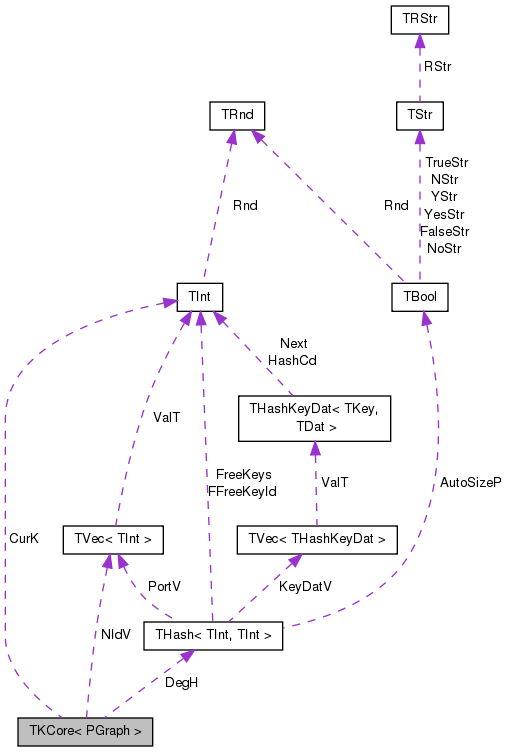[legend]

## Public Member Functions

TKCore (const PGraph &_Graph)

int GetCurK () const

int GetNextCore ()

int GetCoreK (const int &K)

int GetCoreNodes () const
Gets the number of nodes in the K-core (for the current value of K). More...

int GetCoreEdges () const
Gets the number of edges in the K-core (for the current value of K). More...

const TIntVGetNIdV () const
Returns the IDs of the nodes in the current K-core. More...

PGraph GetCoreG () const
Returrns the graph of the current K-core. More...

void Init ()

PGraph Graph

TIntH DegH

TInt CurK

TIntV NIdV

## Detailed Description

### template<class PGraph> class TKCore< PGraph >

K-Core decomposition of a network. K-core is defined as a maximal subgraph of the original graph where every node points to at least K other nodes. K-core is obtained by repeatedly deleting nodes of degree < K from the graph until no nodes of degree < K exist. If the input graph is directed we treat it as undirected multigraph, i.e., we ignore the edge directions but there may be up to two edges between a pair of nodes. See the kcores example (examples/kcores/kcores.cpp) for how to use the code. For example: for (KCore(Graph); KCore.GetNextCore()!=0; ) { } will produce a sequence of K-cores for K=1...

Definition at line 11 of file kcore.h.

## Constructor & Destructor Documentation

template<class PGraph>
 TKCore< PGraph >::TKCore ( const PGraph & _Graph )
inline

Definition at line 20 of file kcore.h.

References TKCore< PGraph >::Init().

20 : Graph(_Graph) { Init(); }
PGraph Graph
Definition: kcore.h:13
void Init()
Definition: kcore.h:43

Here is the call graph for this function: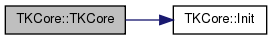## Member Function Documentation

template<class PGraph >
 int TKCore< PGraph >::GetCoreEdges ( ) const

Gets the number of edges in the K-core (for the current value of K).

Definition at line 53 of file kcore.h.

Referenced by TSnap::GetKCoreEdges().

53  {
54  int CoreEdges = 0;
55  for (int k = DegH.FFirstKeyId(); DegH.FNextKeyId(k); ) {
56  CoreEdges += DegH[k];
57  }
58  return CoreEdges/2;
59 }
bool FNextKeyId(int &KeyId) const
Definition: hash.h:478
int FFirstKeyId() const
Definition: hash.h:278
TIntH DegH
Definition: kcore.h:14

Here is the caller graph for this function: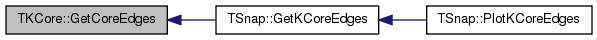template<class PGraph>
 PGraph TKCore< PGraph >::GetCoreG ( ) const
inline

Returrns the graph of the current K-core.

Definition at line 39 of file kcore.h.

References TSnap::GetSubGraph().

39 { return TSnap::GetSubGraph(Graph, NIdV); }
PGraph Graph
Definition: kcore.h:13
TIntV NIdV
Definition: kcore.h:16
PUNGraph GetSubGraph(const PUNGraph &Graph, const TIntV &NIdV, const bool &RenumberNodes)
Returns an induced subgraph of an undirected graph Graph with NIdV nodes with an optional node renumb...
Definition: subgraph.cpp:7

Here is the call graph for this function: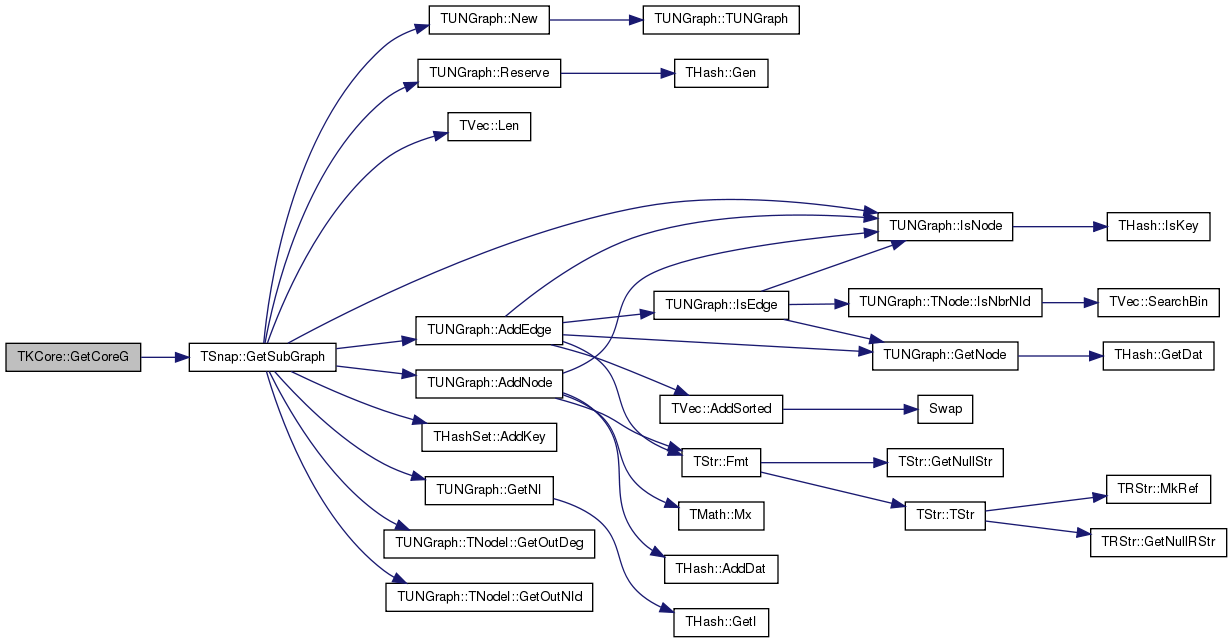template<class PGraph >
 int TKCore< PGraph >::GetCoreK ( const int & K )

Directly generates the core of order K. The function has the same effect as calling GetNextCore() K times.

Definition at line 94 of file kcore.h.

Referenced by TSnap::GetKCore().

94  {
95  Init();
96  CurK = K-1;
97  return GetNextCore();
98 }
TInt CurK
Definition: kcore.h:15
int GetNextCore()
Definition: kcore.h:62
void Init()
Definition: kcore.h:43

Here is the caller graph for this function: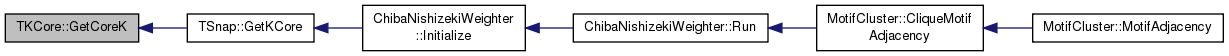template<class PGraph>
 int TKCore< PGraph >::GetCoreNodes ( ) const
inline

Gets the number of nodes in the K-core (for the current value of K).

Definition at line 33 of file kcore.h.

References TVec< TVal, TSizeTy >::Len().

Referenced by TSnap::GetKCoreNodes().

33 { return NIdV.Len(); }
TSizeTy Len() const
Returns the number of elements in the vector.
Definition: ds.h:575
TIntV NIdV
Definition: kcore.h:16

Here is the call graph for this function: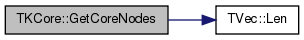Here is the caller graph for this function: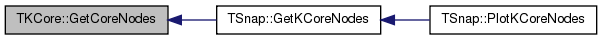template<class PGraph>
 int TKCore< PGraph >::GetCurK ( ) const
inline

Gets the currrent value of K. For every call of GetNextCore() the value of K increases by 1.

Definition at line 23 of file kcore.h.

References TKCore< PGraph >::CurK.

Referenced by TSnap::GetKCoreEdges(), and TSnap::GetKCoreNodes().

23 { return CurK; }
TInt CurK
Definition: kcore.h:15

Here is the caller graph for this function:template<class PGraph >
 int TKCore< PGraph >::GetNextCore ( )

Returns the number of nodes in the next (K=K+1) core. The function starts with K=1-core and every time we call it it increases the value of K by 1 and generates the core. The function proceeds until GetCoreNodes() returns 0. Return value of the function is the size (the number of nodes) in the K-core (for the current value of K).

Definition at line 62 of file kcore.h.

Referenced by TSnap::GetKCoreEdges(), and TSnap::GetKCoreNodes().

62  {
63  TIntV DelV;
64  int NDel=-1, AllDeg=0; //Pass=1;
65  TExeTm ExeTm;
66  CurK++;
67  //printf("Get K-core: %d\n", CurK());
68  while (NDel != 0) {
69  NDel = 0;
70  for (int k = DegH.FFirstKeyId(); DegH.FNextKeyId(k); ) {
71  const int NId = DegH.GetKey(k);
72  const int Deg = DegH[k];
73  if (Deg < CurK) {
74  const typename PGraph::TObj::TNodeI NI = Graph->GetNI(NId);
75  for (int e = 0; e < NI.GetDeg(); e++) {
76  const int n = NI.GetNbrNId(e);
77  const int nk = DegH.GetKeyId(n);
78  if (nk != -1) { DegH[nk] -= 1; } // mark node deleted
79  }
80  DegH.DelKeyId(k);
81  NDel++; AllDeg++;
82  }
83  }
84  //printf("\r pass %d] %d deleted, %d all deleted [%s]", Pass++, NDel, AllDeg, ExeTm.GetStr());
85  }
86  //printf("\n");
87  DegH.Defrag();
88  DegH.GetKeyV(NIdV);
89  NIdV.Sort();
90  return NIdV.Len(); // all nodes in the current core
91 }
TInt CurK
Definition: kcore.h:15
Definition: tm.h:355
TSizeTy Len() const
Returns the number of elements in the vector.
Definition: ds.h:575
PGraph Graph
Definition: kcore.h:13
void DelKeyId(const int &KeyId)
Definition: hash.h:245
void Defrag()
Definition: hash.h:555
void Sort(const bool &Asc=true)
Sorts the elements of the vector.
Definition: ds.h:1318
TIntV NIdV
Definition: kcore.h:16
bool FNextKeyId(int &KeyId) const
Definition: hash.h:478
int FFirstKeyId() const
Definition: hash.h:278
int GetKeyId(const TKey &Key) const
Definition: hash.h:466
void GetKeyV(TVec< TKey > &KeyV) const
Definition: hash.h:484
TIntH DegH
Definition: kcore.h:14
const TKey & GetKey(const int &KeyId) const
Definition: hash.h:252

Here is the caller graph for this function:template<class PGraph>
 const TIntV& TKCore< PGraph >::GetNIdV ( ) const
inline

Returns the IDs of the nodes in the current K-core.

Definition at line 37 of file kcore.h.

References TKCore< PGraph >::NIdV.

Referenced by TSnap::GetKCore().

37 { return NIdV; }
TIntV NIdV
Definition: kcore.h:16

Here is the caller graph for this function: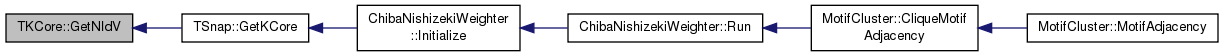template<class PGraph >
 void TKCore< PGraph >::Init ( )
private

Definition at line 43 of file kcore.h.

Referenced by TKCore< PGraph >::TKCore().

43  {
44  DegH.Gen(Graph->GetNodes());
45  for (typename PGraph::TObj::TNodeI NI = Graph->BegNI(); NI < Graph->EndNI(); NI++) {
48  }
49  CurK = 0;
50 }
TInt CurK
Definition: kcore.h:15
PGraph Graph
Definition: kcore.h:13
void Gen(const int &ExpectVals)
Definition: hash.h:222
TIntH DegH
Definition: kcore.h:14
Definition: hash.h:238

Here is the caller graph for this function: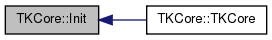## Member Data Documentation

template<class PGraph>
 TInt TKCore< PGraph >::CurK
private

Definition at line 15 of file kcore.h.

Referenced by TKCore< PGraph >::GetCurK().

template<class PGraph>
 TIntH TKCore< PGraph >::DegH
private

Definition at line 14 of file kcore.h.

template<class PGraph>
 PGraph TKCore< PGraph >::Graph
private

Definition at line 13 of file kcore.h.

template<class PGraph>
 TIntV TKCore< PGraph >::NIdV
private

Definition at line 16 of file kcore.h.

Referenced by TKCore< PGraph >::GetNIdV().

The documentation for this class was generated from the following file: